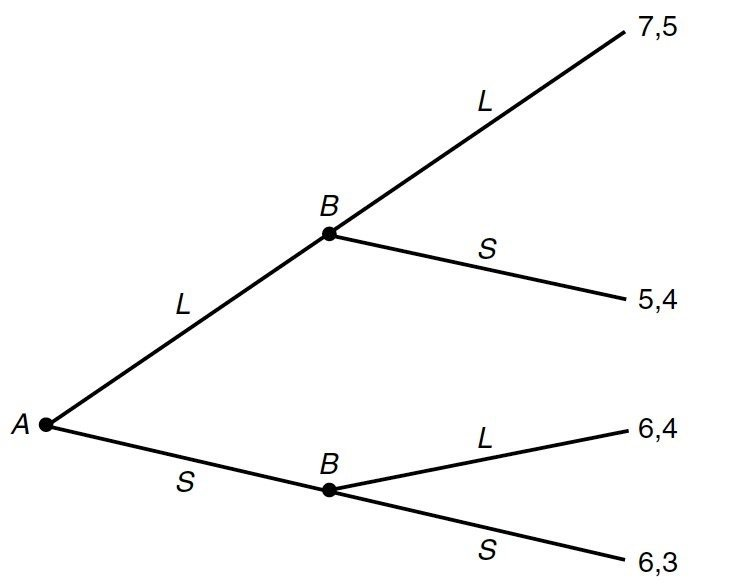# 微观经济学｜博弈论入门

Posted by Derek on March 10, 2019

# 1. 博弈论与博弈The Other Splits The Other Steals One Splits $50075, 50075$ $0, 100150$ One Steals $100150, 0$ $0, 0$

# 2. 纳什均衡

• 若一人认罪并作证检控对方，而对方保持沉默，此人将即时获释，沉默者将判监10年。

• 若两人都保持沉默，则二人同样判监半年。

• 若二人都互相检举，则二人同样判监5年。

 乙沉默 乙背叛 甲沉默 $0.5, 0.5$ $10, 0$ 甲背叛 $0, 10$ $5, 5$

（1）假设已知乙沉默，那么甲一定会背叛；

（2）已知乙背叛，那么甲一定会背叛；

（3）已知甲沉默，那么乙一定会背叛；

（4）已知甲背叛，那么乙一定会背叛。

# 3. 动态博弈

## 3.1 扩展形式的博弈$L, L$ $L, S$ $S, L$ $S, S$ $L$ $7, 5$ $7, 5$ $5, 4$ $5, 4$ $S$ $6, 4$ $6, 3$ $6, 4$ $6, 3$

# Reference

1. 囚徒困境

2. Nicholson, W. and Snyder, C. (2001). Microeconomic Theory. 8th ed. Nashville, TN: South-Western College Pub, 249.

3. Nicholson, W. and Snyder, C. (2001). Microeconomic Theory. 8th ed. Nashville, TN: South-Western College Pub, 256.